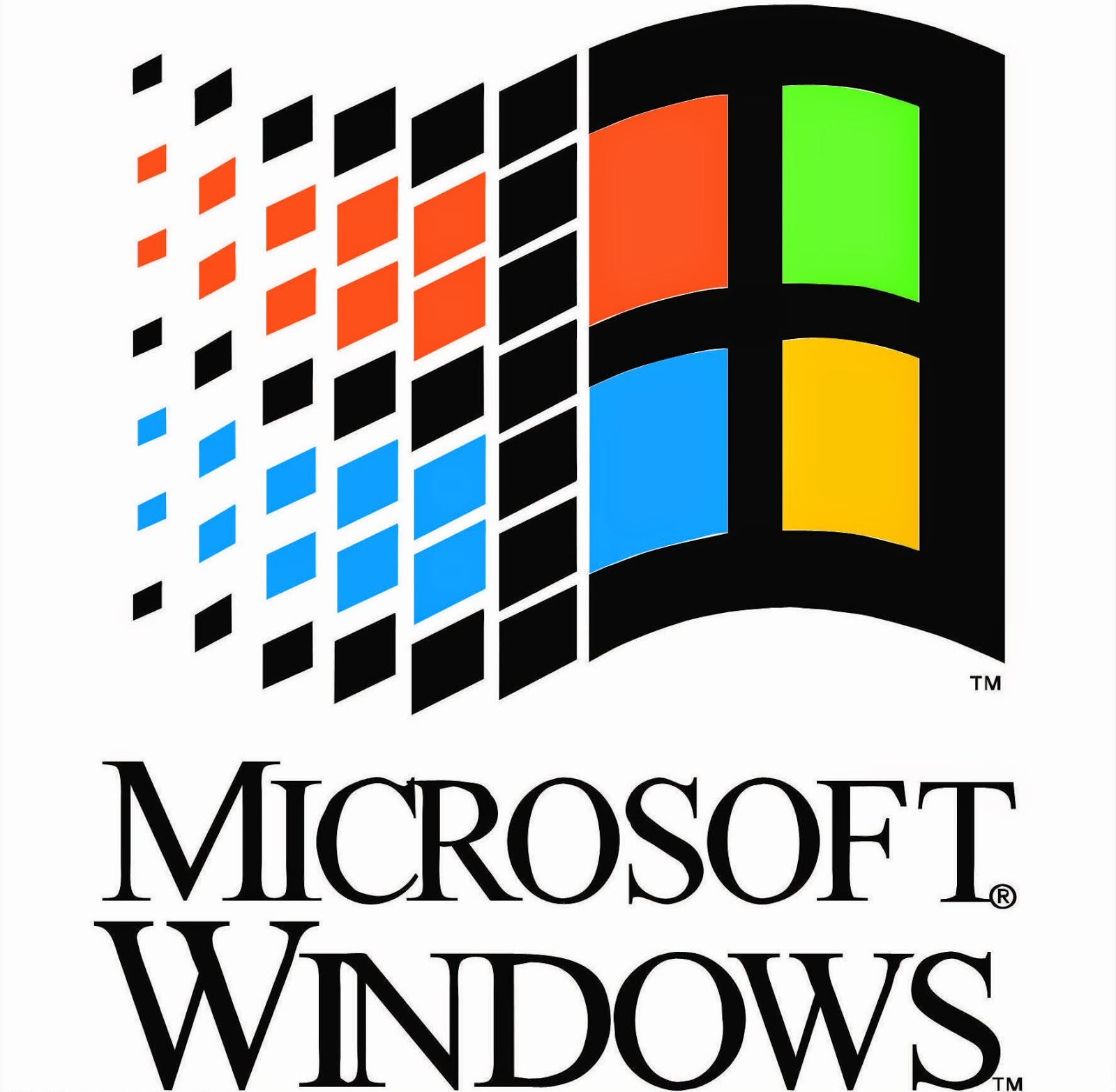## Saturday, June 14, 2014

### 33 | Bill Gates, Agent "33" and Microsoft Monopoly | Illuminati FingerprintsDo you see the "33"?  Look at the black outline around the colored squares.
All this time I have known that Bill Gates is a front for the powers that be.  It isn't he who is the genius he is said to be.  He is just a person, pretending to be someone he is not, much like Mark Zuckerberg.  The Bill and Melinda Gates Foundation is a think tank dedicated to pushing through research that supports corporate and government agendas, no doubt about it.  Their most expensive research to date proved that "classroom size doesn't matter".  Ha!  To a teacher, that is laughable, yet Bill Gates, Mr. "33", spent billions and determined otherwise.
• Bill Gates = 2+9+3+3+7+1+2+5+1 = 33
• Bill = 2+9+3+3 = 17
• Gates = 7+1+2+5+1 = 16
Bill Gates is said to have been born October 28, 1955.
• 10/28/1955 = 1+0+2+8+1+9+5+5 = 31
• Notice the year '55
• Also, October is the only month with "33" numerology
• October = 6+3+2+6+2+5+9 = 33
• 31-33 are the ranging degrees of Masonry
• Masonry = 4+1+1+6+5+9+7 = 33
Side note:  Who knew how much Bill Gates and Kanye West had in common?
• Kanye West = 2+1+5+7+5+5+5+1+2 = 33
Also, notice the name Gates, is built on the root word "Gate".
• Gate = 7+1+20+5 = 33
If you're still not convinced, try this fact.  Bill Gates was in charge of Microsoft for 33-years, before stepping down to hand things over to newly hired Steve Ballmer.  Here is an article courtesy of the Economist commemorating this passing of the torch.

His company Microsoft, also has interesting numerology.
• Microsoft = 13+9+3+18+15+19+15+6+20 = 118
• Microsoft = 4+9+3+9+6+1+6+6+2 = 46
Now if you have ever wondered how Microsoft has the stranglehold that it does on the computer market, wonder no longer.  This is the "33", the powers that be.  We live in their show, and a big part of their show, is Microsoft.  Like I've been saying, Bill Gates is a front, just as most of these other CEOs are.  Think Michael Dell.
• Michael = 4+9+3+8+1+5+3 = 33
• Dell = 4+5+12+12 = 33